# Class 8 Maths chapter 2 Exercise 2.6 Solutions

In this page we have Class 8 Maths chapter 2 Exercise 2.6 Solutions . Hope you like them and do not forget to like , social share and comment at the end of the page.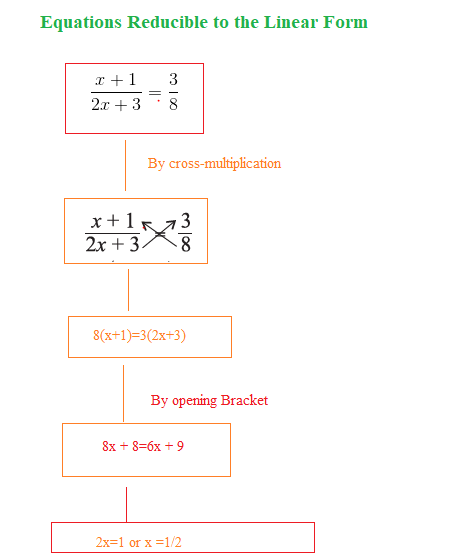## Class 8 Maths chapter 2 Exercise 2.6 Solutions

Question 1
Solve: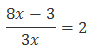On multiplying both sides by 3x, we obtain
8x 3 = 6x
Transposing 6x to LHS and 3 to RHS
8x 6x= 3
2x= 3
Dividing 2 on both the sides
x=3/2

Question 2
Solve: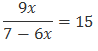On multiplying both sides by 7 6x, we obtain
9x= 15(7 6x)
9x= 105 90x
Transposing 90x on LHS
9x+ 90x= 105
99x= 105
Dividing 99 on both the sides
x=105/99

Question 3
Solve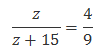On multiplying both sides by 9(z+ 15), we obtain
9z= 4(z+ 15)
9z= 4z+ 60
Transposing 4z on LHS
9z 4z= 60
5z= 60
Dividing 5 on both the sides
z= 12

Question 4
Solve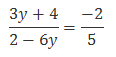On multiplying both sides by 5(2 6y), we obtain
5(3y+ 4) = 2(2 6y)
15y+ 20 = 4 + 12y
Transposing 12y to LHS and 20 to RHS
15y 12y= 4 – 20
3y= 24
Dividing by 3 on both the sides
y= 8

Question 5
Solve: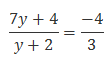On multiplying both sides by 3(y+ 2), we obtain
3(7y+ 4) = 4(y+ 2)
21y+ 12 = 4y - 8
Transposing 4y to LHS and 12 to RHS
21y+ 4y= 8 12
25y= 20
Dividing by 25 on both the sides
y=-20/25=-4/5

Question 6
The ages of Hari and Harry are in the ratio 5:7. Four years from now the ratio of their ages will be 3:4. Find their present ages.
Let the common ratio between their ages bex. Therefore, Hari’s age and Harry’s age will be 5xyears and 7xyears respectively and four years later, their ages will be (5x+ 4) years and (7x+ 4) years respectively.
According to the situation given in the question,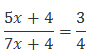Multiplying both the sides by 4(7x+4)
4(5x+4)=3(7x+4)
20x+16=21x+12
Transposing 20x to RHS and 12 to LHS
4=x
x=4
Hari’s age = 5xyears = (5 × 4) years = 20 years
Harry’s age = 7xyears = (7 × 4) years = 28 years
Therefore, Hari’s age and Harry’s age are 20 years and 28 years respectively.

Question 7
The denominator of a rational number is greater than its numerator by 8. If the numerator is increased by 17 and the denominator is decreased by 1, the number obtained is 3/2 . Find the rational number.
Let the numerator of the rational number bex. Therefore, its denominator will
bex+ 8.
The rational number will be x/(x+8) According to the question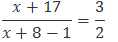2(x+ 17) = 3(x+ 7)
2x+ 34 = 3x+ 21
34 21 = 3x 2x
13 =x
Numerator of the rational number =x= 13
Denominator of the rational number =x+ 8 = 13 + 8 = 21

## Summary

1. Class 8 Maths chapter 2 Exercise 2.6 Solutions has been prepared by Expert with utmost care. If you find any mistake.Please do provide feedback on mail. You can download the solutions as PDF in the below Link also
2. This chapter 2 has total 6 Exercise 2.1,2.2,2.3,2.4,2.5.2.6. This is the Final exercise in the chapter.You can explore previous exercise of this chapter by clicking the link below